SLVS510E July   2006  – March 2015

PRODUCTION DATA.

1. Features
2. Applications
3. Description
4. Typical Application Circuit
5. Revision History
6. Device Comparison Table
7. Pin Configuration and Functions
8. Specifications
9. Parameter Measurement Information
10. 10Detailed Description
1. 10.1 Overview
2. 10.2 Functional Block Diagram
3. 10.3 Feature Description
1. 10.3.1 Controller Circuit
4. 10.4 Device Functional Modes
11. 11Application and Implementation
1. 11.1 Application Information
2. 11.2 Typical Application
1. 11.2.1 Design Requirements
2. 11.2.2 Detailed Design Procedure
3. 11.2.3 Application Curves
3. 11.3 System Examples
12. 12Power Supply Recommendations
13. 13Layout
14. 14Device and Documentation Support
15. 15Mechanical, Packaging, and Orderable Information

#### Package Options

Refer to the PDF data sheet for device specific package drawings

• DDC|6

## 11 Application and Implementation

NOTE

Information in the following applications sections is not part of the TI component specification, and TI does not warrant its accuracy or completeness. TI’s customers are responsible for determining suitability of components for their purposes. Customers should validate and test their design implementation to confirm system functionality.

### 11.1 Application Information

The TPS6107x DC-DC converters are intended for systems powered by a single-cell, up to triple-cell alkaline, NiCd, NiMH battery with a typical terminal voltage between 0.9 V and 5.5 V. They can also be used in systems powered by one-cell Li-Ion or Li-Polymer with a typical voltage between 2.5 V and 4.2 V. Additionally, any other voltage source with a typical output voltage between 0.9 V and 5.5 V can power systems where the TPS6107x is used. Due to the nature of boost converters, the output voltage regulation is only maintained when the input voltage applied is lower than the programmed output voltage.

### 11.2 Typical Application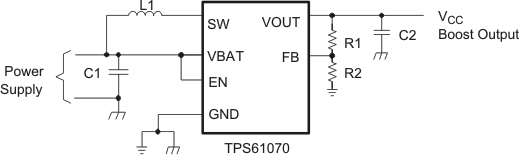Figure 12. Typical Application Circuit for Adjustable Output Voltage Option

#### 11.2.1 Design Requirements

In this example, TPS61070 is used to design a 3.3-V power supply with 75-mA output current capability. The TPS61200 can be powered by either a single-cell, two-cell, or three-cell alkaline, NiCd or NiMH, or one-cell Li-Ion or Li-Polymer battery. In this example, the input voltage range is from 0.9 V to 1.65 V for single-cell alkaline input design.

#### 11.2.2.1 Programming the Output Voltage

The output voltage of the TPS6107x dc/dc converter can be adjusted with an external resistor divider. The typical value of the voltage at the FB pin is 500 mV. The maximum recommended value for the output voltage is 5.5 V. The current through the resistive divider should be about 100 times greater than the current into the FB pin. The typical current into the FB pin is 0.01 µA, and the voltage across R2 is typically 500 mV. Based on those two values, the recommended value for R2 should be lower than 500 kΩ, in order to set the divider current at 1 µA or higher. Because of internal compensation circuitry, the value for this resistor should be in the range of 200 kΩ. From that, the value of resistor R1, depending on the needed output voltage (VO), is calculated using Equation 1:

Equation 1.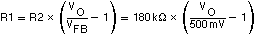For example, if an output voltage of 3.3 V is needed, a 1 MΩ resistor should be chosen for R1. If for any reason the value chosen for R2 is significantly lower than 200 kΩ, additional capacitance in parallel to R1 is recommended, if the device shows instable regulation of the output voltage. The required capacitance value is calculated using Equation 2:

Equation 2.#### 11.2.2.2 Inductor Selection

A boost converter normally requires two main passive components for storing energy during the conversion. A boost inductor and a storage capacitor at the output are required. To select the boost inductor, it is recommended to keep the possible peak inductor current below the current limit threshold of the power switch in the chosen configuration. For example, the current limit threshold of the TPS6107x's switch is 600 mA. The highest peak current through the inductor and the switch depends on the output load, the input (VBAT), and the output voltage (VOUT). Estimation of the maximum average inductor current is done using Equation 3:

Equation 3.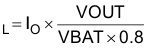For example, for an output current of 75 mA at 3.3 V, at least 340 mA of average current flows through the inductor at a minimum input voltage of 0.9 V.

The second parameter for choosing the inductor is the desired current ripple in the inductor. Normally, it is advisable to work with a ripple of less than 20% of the average inductor current. A smaller ripple reduces the magnetic hysteresis losses in the inductor, as well as output voltage ripple and EMI. But in the same way, regulation time rises at load changes. In addition, a larger inductor increases the total system costs. With these parameters, it is possible to calculate the value for the inductor by using Equation 4:

Equation 4.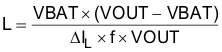Parameter f is the switching frequency and ΔIL is the ripple current in the inductor, i.e., 40% ΔIL. In this example, the desired inductor has the value of 4 µH. With this calculated value and the calculated currents, it is possible to choose a suitable inductor. In typical applications, a 4.7-µH inductance is recommended. The device has been optimized to operate with inductance values between 2.2 µH and 10 µH. Nevertheless, operation with higher inductance values may be possible in some applications. Detailed stability analysis is then recommended. Care must be taken because load transients and losses in the circuit can lead to higher currents as estimated in Equation 4. Also, the losses in the inductor caused by magnetic hysteresis losses and copper losses are a major parameter for total circuit efficiency.

The following inductor series from different suppliers have been used with the TPS6107x converters:

### Table 1. List of Inductors

VENDOR INDUCTOR SERIES
TDK VLF3010
VLF4012
Wurth Elektronik 744031xxx
744042xxx
EPCOS B82462-G4
Cooper Electronics Technologies SD18
SD20
Taiyo Yuden CB2016B xxx
CB2518B xxx

#### 11.2.2.3.1 Input Capacitor

At least a 10 µF input capacitor is recommended to improve transient behavior of the regulator and EMI behavior of the total power supply circuit. A ceramic capacitor or a tantalum capacitor with a 100-nF ceramic capacitor in parallel, placed close to the IC, is recommended.

#### 11.2.2.3.2 Output Capacitor

The major parameter necessary to define the output capacitor is the maximum allowed output voltage ripple of the converter. This ripple is determined by two parameters of the capacitor, the capacitance and the ESR. It is possible to calculate the minimum capacitance needed for the defined ripple, supposing that the ESR is zero, by using Equation 5:

Equation 5.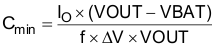Parameter f is the switching frequency and ΔV is the maximum allowed ripple.

With a chosen ripple voltage of 10 mV, a minimum capacitance of 4.5 µF is needed. In this value range, ceramic capacitors are a good choice. The ESR and the additional ripple created are negligible. It is calculated using Equation 6:

Equation 6.The total ripple is the sum of the ripple caused by the capacitance and the ripple caused by the ESR of the capacitor. Additional ripple is caused by load transients. This means that the output capacitor has to completely supply the load during the charging phase of the inductor. The value of the output capacitance depends on the speed of the load transients and the load current during the load change. With the calculated minimum value of 4.5 µF and load transient considerations, the recommended output capacitance value is in a 10 µF range.

Care must be taken on capacitance loss caused by derating due to the applied dc voltage and the frequency characteristic of the capacitor. For example, larger form factor capacitors (in 1206 size) have their self resonant frequencies in the same frequency range as the TPS6107x operating frequency. So the effective capacitance of the capacitors used may be significantly lower. Therefore, the recommendation is to use smaller capacitors in parallel instead of one larger capacitor.

#### 11.2.2.4 Small Signal Stability

To analyze small signal stability in more detail, the small signal transfer function of the error amplifier and the regulator, which is given in Equation 7, can be used:

Equation 7.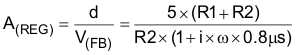#### 11.2.3 Application Curves

FIGURE
Output voltage in continuous mode (TPS61071) Figure 13
Output voltage in continuous mode (TPS61071) Figure 14
Output voltage in power-save mode (TPS61070) Figure 15
Output voltage in power-save mode (TPS61070) Figure 16
Load transient response (TPS61071) Figure 17
Load transient response (TPS61071) Figure 18
Line transient response (TPS61071) Figure 19
Line transient response (TPS61071) Figure 20
Start-up after enable (TPS61070) Figure 21
Start-up after enable (TPS61070) Figure 22
Start-up after enable (TPS61071) Figure 23
Start-up after enable (TPS61071) Figure 24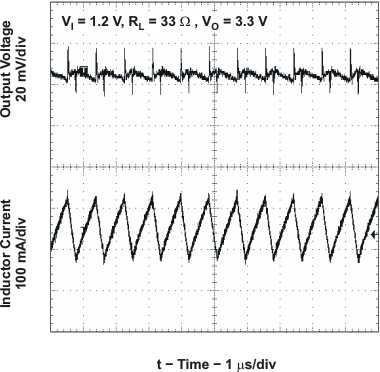Figure 13. TPS61071 Output Voltage in Continuous Mode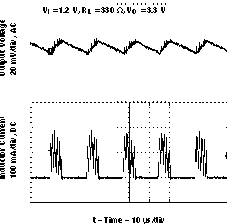Figure 15. TPS61070 Output Voltage in Power-Save Mode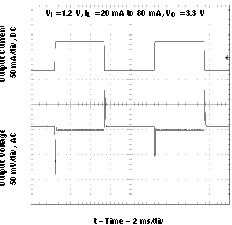Figure 17. TPS61071 Load Transient Response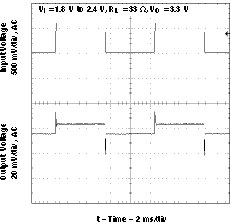Figure 19. TPS61071 Line Transient Response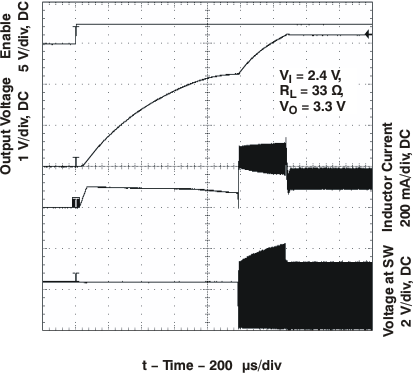Figure 21. TPS61070 Start-Up After Enable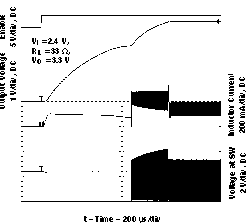Figure 23. TPS61071 Start-Up After Enable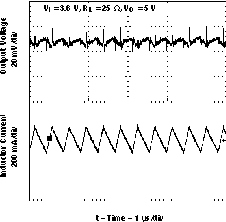Figure 14. TPS61071 Output Voltage In Continuous Mode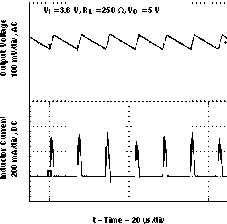Figure 16. TPS61070 Output Voltage in Power-Save Mode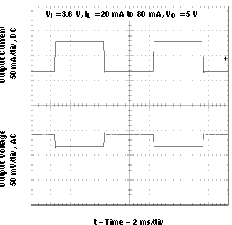Figure 18. TPS61071 Load Transient Response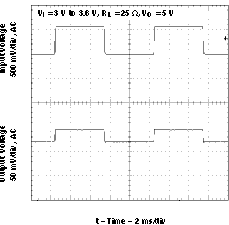Figure 20. TPS61071 Line Transient ResponseFigure 22. TPS61070 Start-Up After Enable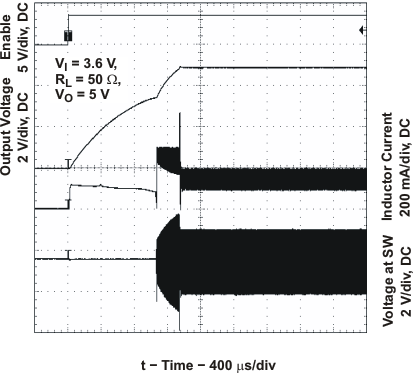Figure 24. TPS61071 Start-Up After Enable

### 11.3 System Examples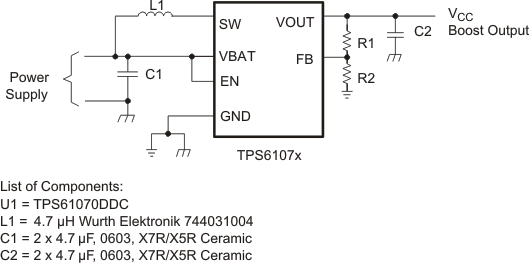Figure 25. Power Supply Solution for Maximum Output Power Operating from a Single or
Dual Alkaline CellFigure 26. Power Supply Solution Having Small Total Solution Size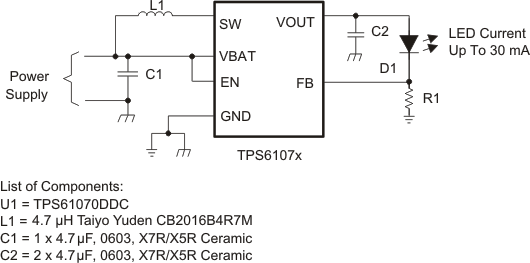Figure 27. Power Supply Solution for Powering White LEDs in Lighting Applications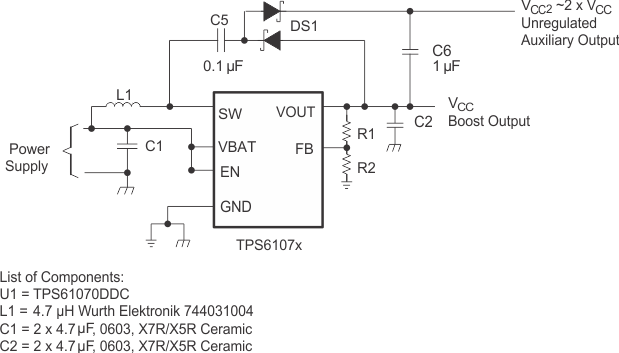Figure 28. Power Supply Solution With Auxiliary Positive Output Voltage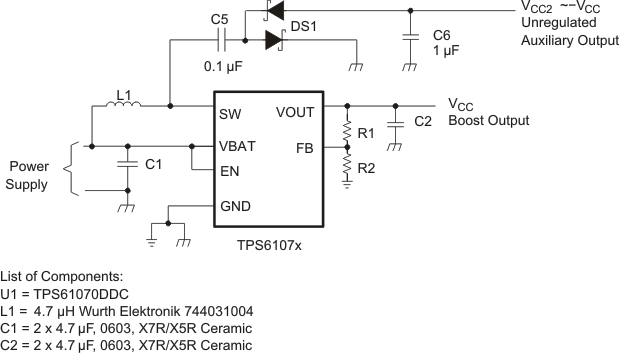Figure 29. Power Supply Solution With Auxiliary Negative Output Voltage